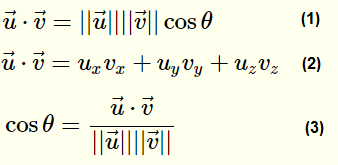# Calculating the angle between two polygons

For my application, I want to render models as polygonal surfaces in 3D space and have edges that are bent "outwards" to have a solid line and those who are bent "inwards" to have a dotted line. So if I look from the top of the edge if they "bend towards me" they are bending inwards, if they bend away from me, they bend outwards.

For this, I need to calculate the angle between two connected triangles. How can I calculate this angle? How can I define if it is bent outwards or inwards (looking from the top it would always bend downwards if the angle is >180 and inwards (so up) if the angle is <180, but how can I calculate this?

• You might have to give a little more context here. I think I know what you're talking about but it's a little fuzzy. For example, are we talking about polygons in 3D-space here? Are they planar (though, it seems they are when you're concentrating on triangles)? When you say "looking from the top", do you actually mean "looking from the side"? It seems you're talking about the angle between adjacent triangles of a triangular mesh in 3D space, but I'm not entirely sure and a little more clarity and accuracy in the question's wording might be useful. – Christian Rau Sep 15 '19 at 16:06
• I'm sorry for the unclear question. It is in 3D space. The triangles are planar. 2 Triangles always share one common edge. For this edge I want to know if it is bent outwards or inwards. So if i look from the top of the edge if they "bend towards me" they are bending inwards, if they bend away from me, they bend outwards. Does this make sense? edit: maybe instead looking from the top, it is better to say that I look from the outside of the two triangles (their front faces) – Anima Sep 15 '19 at 16:09
• Yes, that helps. I tried to extend the question a little. – Christian Rau Sep 15 '19 at 16:11

I suggest computing the normal vector for each polygon and compute the angle between the normal vector of one triangle and the normal vector of a plane perpendicular to the other surface that passes through the common edge.

See the following illustration, where the red arrows are the normal vectors of the red polygons. The blue vector is the plane perpendicular to the left polygon that passes through the edge common to both polygons.If your polygons are planar, then you can compute the normal for any polygon ABC by computing the cross product AB x AC.

To obtain the blue vector, take the cross product of the normal of the left polygon and the vector that is the common edge.

The angle you're looking for is formed between this blue vector and the normal to the other polygon (the red vector on the right in the diagram).

You then compute the cosine of the angle between two vectors u and v by taking their dot product, then dividing by their magnitudes.Image taken from here.

• I don’t think this approach will quite work here. Since the dot product is symmetrical around 0°, it’ll have the same value whether the normals are pointing “slightly towards” or “slightly away” from each other. The blue stuff in your diagram is relevant, but it’d be worth explaining how to get that vector. – Noah Witherspoon Sep 16 '19 at 18:06
• You're right. Sorry. To get the blue vector you take the cross product of the normal vector and the common edge. Then you compare the blue vector and the other red vector. I'll update the answer. – Wyck Sep 16 '19 at 18:11
• @NoahWitherspoon I've fixed it. Thanks very much for pointing out that rather glaring omission. – Wyck Sep 16 '19 at 18:19
• While likely clear to the inclined geometry enthusiast, it's probably worth noting that direction very much matters here (as it usually does in a cross product), otherwise nothing stops me from using the common edge vector the "wrong" way around (or knowing which way is the "right" one to begin with) and basically again sitting there without knowing if the angle is the right way around. I mean, the approach is good and works, but it can't hurt to explain what's actually going on here. – Christian Rau Sep 16 '19 at 22:16
• @ChristianRau The expected behaviour is most likely consistent with the normals used for back/front face culling, so the direction is already defined by the order in which the vertices are given. – lightxbulb Sep 17 '19 at 7:04